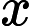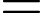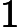# Sets and their Representations Questions and Answers [Set MCQs and Answers]

These Sets and their Representations Questions and Answers are frequently asked in various exams including IIT-JEE/ JEE-Mains as we as GATE exam also. These are some selective Sets and their Representations Multiple Choice Questions and Answers, that will help you most.

## Sets and their Representations Questions and Answers

#### 1. Write the set 1/2,2/3,3/4,4/5,5/6 in set builder form.

a) {x: x=n/n+1 where n is a natural number less than 6}
b) {x: x=n+1/n+2 where n is a natural number less than 6}
c) {x: x=n+1/n where n is a natural number less than 6}
d) {x: x=n/n+1 where n is a natural number less than 5}

Explanation: Since the denominator is one more than the numerator and only 5 terms are present. So, the above set can be written as {x:where n is a natural number less than 6}.

#### 2. Which of the following set is not possible?

a) Honest persons
b) Prime numbers up to 100
c) Even numbers up to 100
d) Letters forming the word SCHOOL

Explanation: A set is a collection of well-defined objects but honesty has no precise definition.
A prime number is a number that can be divisible only by 1 and itself.
Even a number is a number that can be divisible by 2.
Set of letters forming the word SCHOOL {S,C,H,O,L}.

#### 3. Write solution set of equation x2-3x+2=0 in roster form.

a) {1,3}
b) {2,4}
c) {1,4}
d) {1,2}

Explanation: x2-3x+2=0
=>x2-x-2x-2=0=>x(x-1)-2(x-1)=0
=>(x-1)(x-2)=0=>x=1,2
so, the solution set of equation x2-3x+2=0 in roster form is {1,2}.

#### 4. Write the set {x : x is an integer and x2-9=0} in roster form.

a) {3}
b) {-3}
c) {3,-3}
d) {9,3}

Explanation: Since x is given as an integer so x can be positive as well as negative.
x2-9=0 => (x-3)(x+3)=0 => x=3,-3.
So, the set {x : x is an integer and x2-9=0} can be written as {3,-3}.

#### 5. Write the set {x : x is a natural number and x2-9=0} in roster form.

a) {3}
b) {-3}
c) {3,-3}
d) {9,3}

Explanation: Since x is given as natural number so x can be positive only. x2-9=0 => (x-3)(x+3)=0 => x=3,-3.
Here, -3 is not a natural number so, the set {x : x is a natural number and x2-9=0} can be written as {3}.

#### 6. Let A={1,2,3,4,5}. Insert appropriate symbol in 2 ________ A.

a) =
b) <
c) ∈
d) ∉

Explanation: Here, 2 is an element of set A.
So, 2 belong to set A. 2∈A.

#### 7. Let X={1,2,3,4,5,6}. Insert appropriate symbol in 9 ________ X.

a) =
b) <
c) ∈
d) ∉

Explanation: Here, 9 is not an element of set X.
So, 9 does not belong to set A. 9∉X.

#### 8. Which of the following does not belong to set {x : x is a vowel in English alphabet}?

a) e
b) b
c) i
d) o

Explanation: There are five vowels in English Alphabet a, e, i, o, u. So, set can be written in roster form as {a, e, i, o, u}. ‘b’ does not belongs to given set.

#### 9. The number of elements in set {x : x is a letter of word TRIGONOMETRY} is __________

a) 8
b) 7
c) 9
d) 10

Explanation: The above set can be represented as {T,R,I,G,O,N,M,E,Y}. So, the number of elements in the set is 9.

#### 10. What is the solution set of the equation X2+3X+2=0 in roster form?

a) {-1, 2}
b) {-1, -2}
c) {1, -2}
d) {1, 2}

Explanation: Solving the equation:
X2+2X+X+2=0
(x+2) (X+1) = 0
X= -2 and X = -1

#### 11. Which one of the following is the correct representation for the set {x: x is a positive integer and x3<50} in roster form?

a) {0,1,2,3,4,5}
b) {-1,1,2,3}
c) {1,2,3}
d) {0,1,2,3}

Explanation: 0 is not a positive integer moreover
33<50 and 43>50

#### 12. Which one of the following is the correct representation of set A = {1,3,5,7….} in set builder form?

a) {x: x = 2n where n ∈ N}
b) {x: x = n2-1 where n ∈ N}
c) {x: x = 2n+1 where n ∈ N}
d) {x: x = 2n-1 where n ∈ N}

Explanation: The given sequence is an odd number sequence of the format 2n+1 or 2n-1, but since n is given as a natural number so 2n+1 is not valid as 0 is not a natural number hence 2n-1 is the correct answer.

a) 0 ∈ A
b) 2 ∈ A
c) 3 ∉ A
d) 5 ∉ A